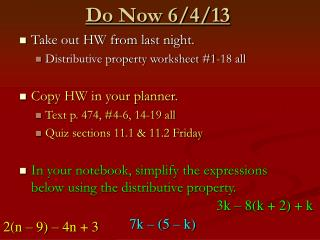DownloadDownload PresentationDo Now 6/4/13

# Do Now 6/4/13

Télécharger la présentation## Do Now 6/4/13

- - - - - - - - - - - - - - - - - - - - - - - - - - - E N D - - - - - - - - - - - - - - - - - - - - - - - - - - -
##### Presentation Transcript

1. Do Now 6/4/13 • Take out HW from last night. • Distributive property worksheet #1-18 all • Copy HW in your planner. • Text p. 474, #4-6, 14-19 all • Quiz sections 11.1 & 11.2 Friday • In your notebook, simplify the expressions below using the distributive property. 3k – 8(k + 2) + k 7k – (5 – k) 2(n – 9) – 4n + 3

2. 1) 6c + 66 2) 28 – 7k 3) -44 + (-11x) 4) 35 + (-5r) 5) -14 + (-6y) 6) 10 – 2p 7) 6b – 102 8) -40c² + (-20) 9) 8 – 18p 10) –x + (-10) 11) -21 + 6p 12) -9 + f 13) 10y + 12 14) 45y + (-27) 15) 5x + 14 16) 32x + (-40) 17) -17w + (-1) 18) -14j + 3 HomeworkPractice worksheet #1-18

3. Simplifying an Expression withthe Distributive Property 2(n – 9) – 4n + 3 Write expression as a sum “Opp-Opp” 2(n + (-9)) + (-4n) + 3 2n + (-18) + (-4n) + 3 Distributive Property 2n + (-4n) + (-18) + 3 Commutative Property COLLECT LIKE TERMS -2n + (-15) or -2n – 15

4. Simplifying an Expression withthe Distributive Property 3k – 8(k + 2) + k Write expression as a sum “Opp-Opp” 3k + (-8)(1k + 2) + 1k 3k + (-8k) + (-16) + 1k Distributive Property 3k + (-8k) + 1k + (-16) Commutative Property COLLECT LIKE TERMS -4k + (-16) or -4k – 16

5. Place a ‘1’ in front of the parentheses when you have a subtraction sign. Simplifying an Expression withthe Distributive Property 7k – (5 – k) 7k – 1(5 – k) Write expression as a sum “Opp-Opp” 7k + (-1)(5 + (-1k)) 7k + (-5) +1k Distributive Property 7k + 1k + (-5) Commutative Property COLLECT LIKE TERMS 8k + (-5) 8k – 5 or

6. Objective • SWBAT solve multi-step equations having like terms AND the distributive property

7. Equation- • mathematical sentence with an equal sign Like a scale, both sides must be EQUAL in order to be balanced.

8. Section 11.2 “Solving a Multi-Step Equation” How can you get the “unknown” by itself?

9. Solving Multi-Step Equations… Isolate the variable! Get ‘x’ by itself. 10 + 3(x + 2) = 31 10 + 3x + 6 = 31 Distributive Property 10 + 6 + 3x = 31 Combine like terms 16 + 3x = 31 - 16 -16 “Undo” addition 3x = 15 3 3 “Undo” multiplication x = 5

10. Check Your Work! 10 + 3(x + 2) = 31 x = 5 10 + 3((5) + 2) = 31 Are both sides equal?

11. -2(x – 6) + 7 = 35 “Opp-Opp” -2(x + (-6)) + 7 = 35 -2x + 12 + 7 = 35 Distributive Property Combine like terms -2x + 19 = 35 -19 -19 “Undo” addition -2x = 16 “Undo” multiplication -2 -2 x = -8

12. -20 – (4x – 1) = -15 “Opp-Opp” -20 + (-1)(4x + (-1)) = -15 -20 + (-4x) + 1 = -15 Distributive Property Combine like terms -19 + (-4x) = -15 +19 +19 “Undo” addition -4x = 4 “Undo” multiplication -4 -4 x = -1

13. Guided Practice 12(x + 3) – 3x = 117 -25 + 4(2x + 5) = -61 12x – 3x + 36 = 117 12x + 36 – 3x = 117 -25 + 8x + 20 = -61 -25 + 20 +8x = -61 9x + 36 = 117 -5 + 8x = -61 – 36 –36 +5 + 5 9x = 81 8x = -56 9 9 8 8 x = 9 x = -7

14. Homework 24 5 18 17 • Text p. 474, #4-6, 14-19 all 2